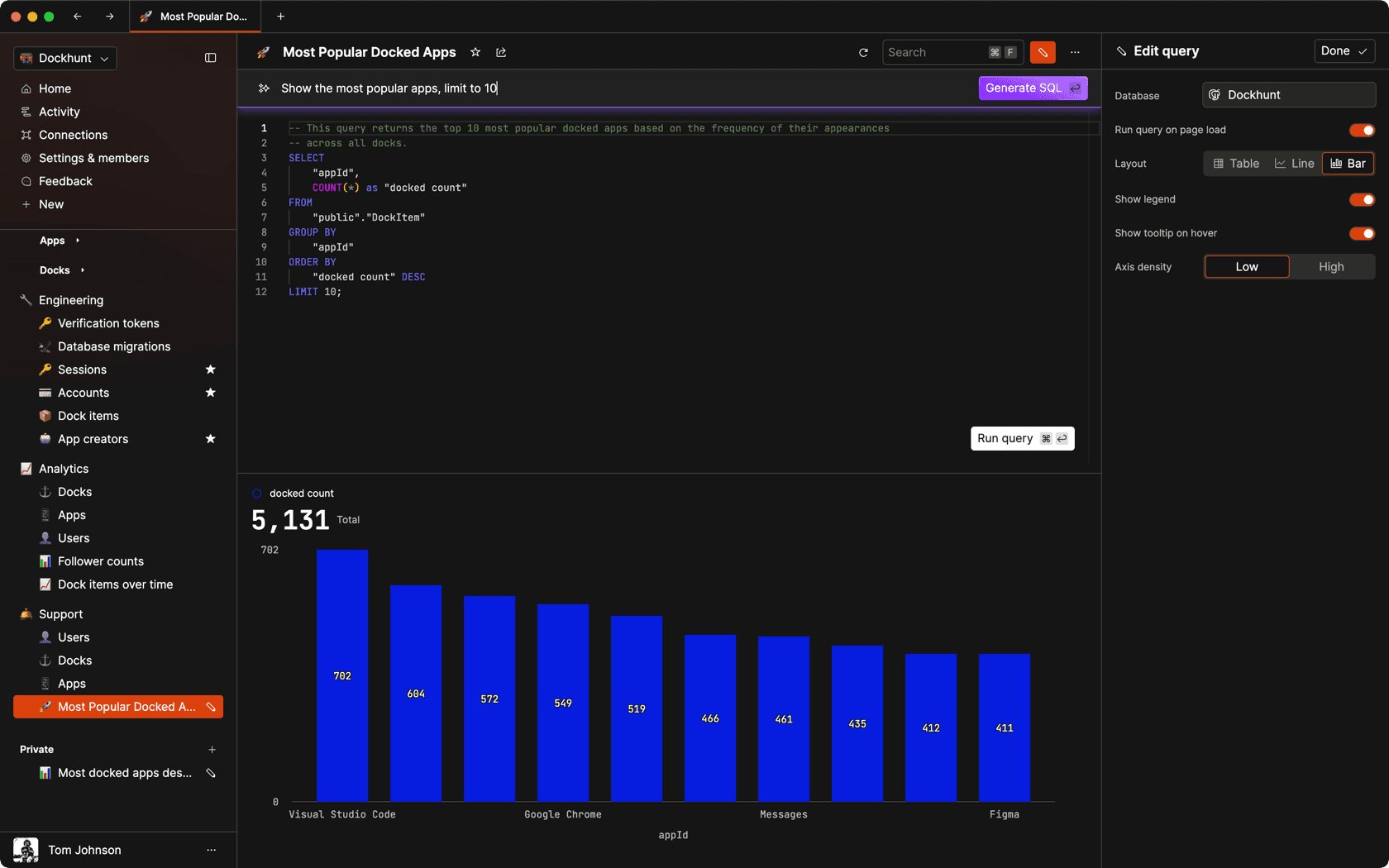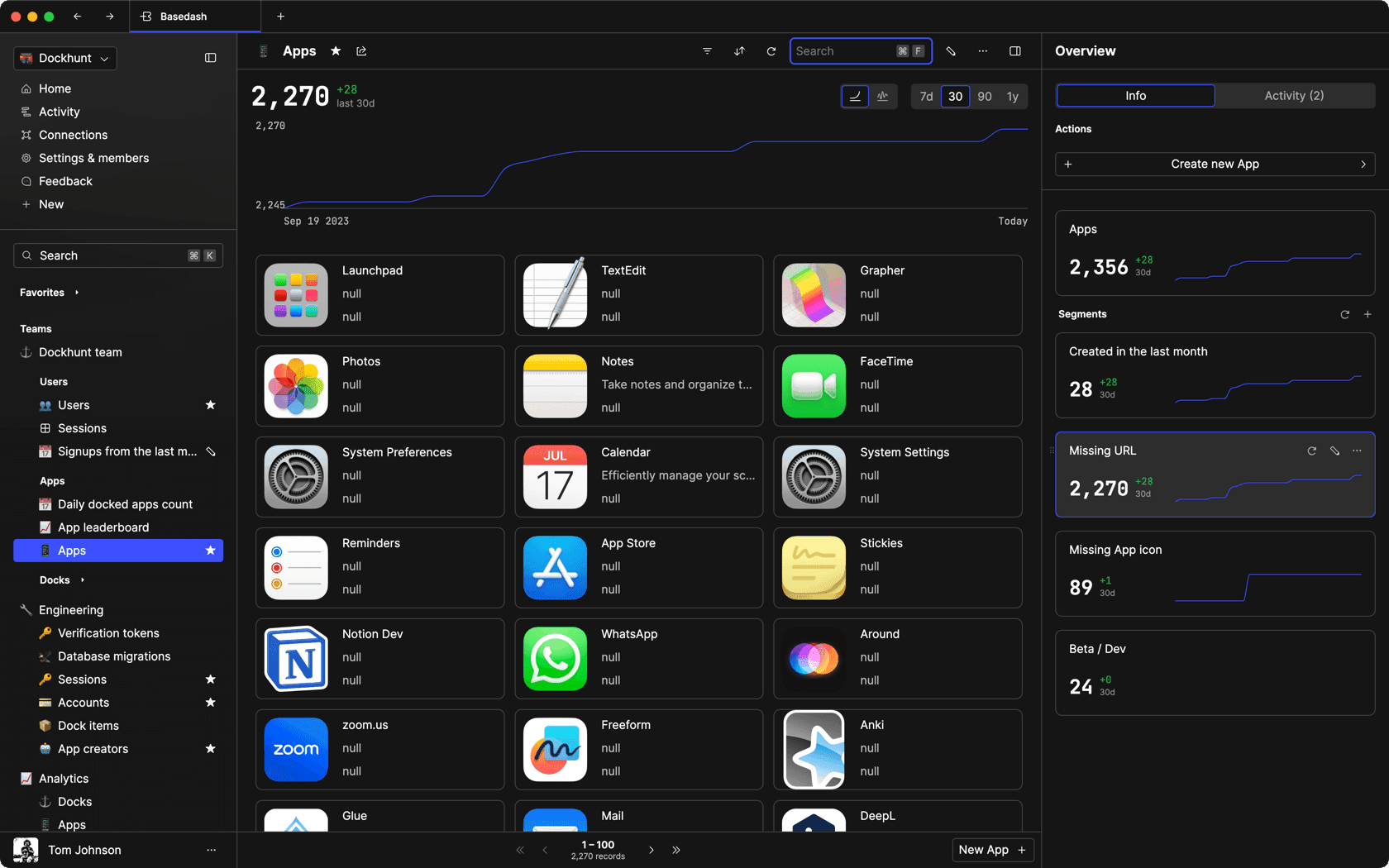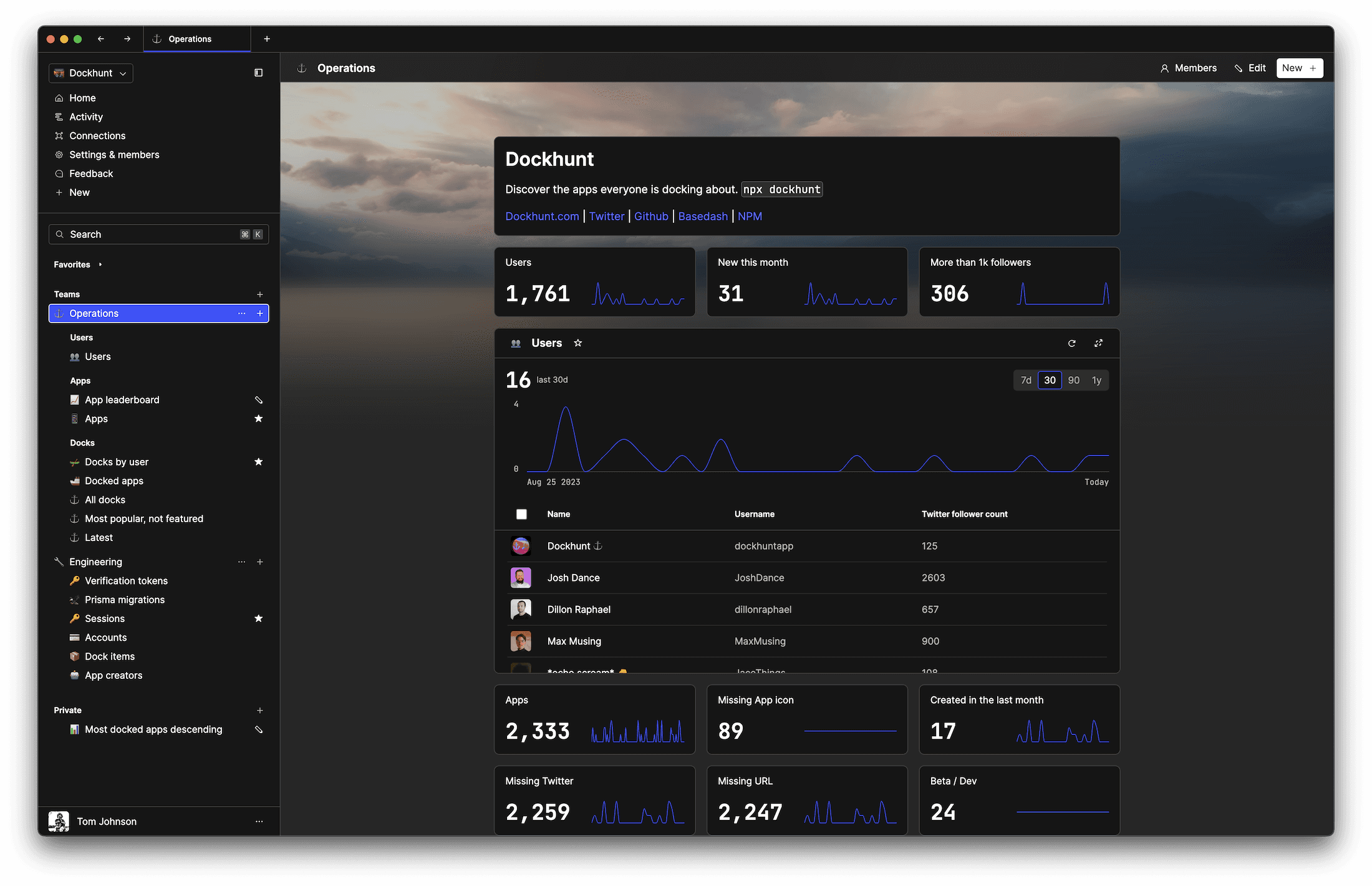# Bubble sort in JavaScriptself.__wrap_n=self.__wrap_n||(self.CSS&&CSS.supports("text-wrap","balance")?1:2);self.__wrap_b=(e,r,t)=>{t=t||document.querySelector(`[data-br="\${e}"]`);let n=t.parentElement,a=s=>t.style.maxWidth=s+"px";t.style.maxWidth="";let o,i=n.clientWidth,l=n.clientHeight,c=i/2-.25,f=i+.5;if(i){for(a(c),c=Math.max(t.scrollWidth,c);c+1<f;)o=Math.round((c+f)/2),a(o),n.clientHeight===l?f=o:c=o;a(f*r+i*(1-r))}t.__wrap_o||typeof ResizeObserver<"u"&&(t.__wrap_o=new ResizeObserver(()=>{self.__wrap_b(0,+t.dataset.brr,t)})).observe(n)};self.__wrap_n!=1&&self.__wrap_b(":Rj0pm:",1)

November 6, 2023

Bubble sort is a simple sorting algorithm that repeatedly steps through the list, compares adjacent elements, and swaps them if they are in the wrong order. The pass-through is repeated until no swaps are needed, which indicates that the list is sorted. We’ll go into more detail on this below.

## How bubble sort works

The algorithm works by comparing each pair of adjacent items and swapping them if they are in the wrong order. This process continues through the array until no swaps are required, indicating that the array is sorted. The algorithm gets its name because smaller elements "bubble" to the top of the list.

## Detailed logic behind bubble sort

In bubble sort, the invariant is that at the end of each pass, the largest element among the unsorted elements is moved to its correct position. This results in elements "bubbling up" like a bubble in water to their rightful place, hence the name. Here's a breakdown of the process:

2. If the first element is greater than the second, swap them.
3. Move to the next pair of elements and repeat the comparison and swap if necessary.
4. Continue this process until the end of the array is reached, which places the largest element in its final position.
5. Repeat the entire process for the remaining elements, excluding the last sorted elements.
6. The algorithm terminates when a pass through the array requires no swaps, meaning the array is sorted.

## The bubble sort algorithm in js

```function bubbleSort(arr) {
let len = arr.length;
let swapped;
do {
swapped = false;
for (let i = 0; i < len - 1; i++) {
if (arr[i] > arr[i + 1]) {
let temp = arr[i];
arr[i] = arr[i + 1];
arr[i + 1] = temp;
swapped = true;
}
}
} while (swapped);
return arr;
}```

## Optimizing bubble sort

A small optimization to the standard bubble sort algorithm is to reduce the number of items checked each pass since the last item of the previous pass is already in place:

```function optimizedBubbleSort(arr) {
let len = arr.length;
for (let i = 0; i < len; i++) {
for (let j = 0; j < len - i - 1; j++) {
if (arr[j] > arr[j + 1]) {
// Swap elements
[arr[j], arr[j + 1]] = [arr[j + 1], arr[j]];
}
}
}
return arr;
}```

To improve the best-case performance, we can introduce an adaptive change to the bubble sort algorithm by adding a flag that checks whether any swaps were made in the inner loop:

```function adaptiveBubbleSort(arr) {
let len = arr.length;
let swapped;
do {
swapped = false;
for (let i = 0; i < len - 1; i++) {
if (arr[i] > arr[i + 1]) {
[arr[i], arr[i + 1]] = [arr[i + 1], arr[i]];
swapped = true;
}
}
len--; // Decrease the length to avoid unnecessary comparisons
} while (swapped);
return arr;
}```

With this improvement, if during a pass no swaps are made, the algorithm concludes that the list must be sorted and stops early, thereby improving efficiency.

## Writing clean and concise bubble sort

For clean and concise code, modern JavaScript offers destructuring assignments that can make swapping elements more readable:

```function bubbleSortClean(arr) {
for (let i = 0; i < arr.length; i++) {
for (let j = 0; j < arr.length - i - 1; j++) {
if (arr[j] > arr[j + 1]) {
[arr[j], arr[j + 1]] = [arr[j + 1], arr[j]]; // Swapping using destructuring
}
}
}
return arr;
}```

## Performance considerations

Bubble sort is not suitable for large datasets as its average and worst-case complexity are both O(n^2), where n is the number of items being sorted. There are more efficient algorithms like quicksort, mergesort, or heapsort for larger datasets.

## When to use bubble sort

Bubble sort can be a good choice when simplicity is paramount, or when the list is already mostly sorted, as it has a best-case complexity of O(n) when the list is already sorted.

## Example usage of bubble sort

To see bubble sort in action, you can use the following source code to sort an array and then print it out:

```// Array to be sorted
let numbers = [5, 3, 8, 4, 6];

// Sorting the array
let sortedNumbers = bubbleSort(numbers);

// Output the sorted array
console.log(sortedNumbers);```

## Common pitfalls and how to avoid them

• Inefficiency on large lists: Since bubble sort has a quadratic time complexity, it's inefficient for large lists. Use more efficient algorithms for sorting large datasets.
• Misunderstanding the algorithm: Ensure you comprehend the inner and outer loop logic to avoid incorrect implementations.
• Mutating the original array: If you don't want to mutate the original array, you should make a copy of it before sorting.

## Visualizing bubble sort

Providing visual representation helps to understand the sorting mechanism of bubble sort. Visual aids like animations or step-by-step illustrations show the process of elements being compared and swapped, emphasizing the "bubbling" effect of the larger elements moving to the top.

## Comparing with JavaScript’s built-in sort method

It's beneficial to compare bubble sort with JavaScript’s `Array.prototype.sort()` method, which is much more efficient for most cases. While `Array.prototype.sort()` is a high-level built-in function that works well with all data types and sorts using an advanced algorithm (like quicksort or mergesort depending on the browser), bubble sort serves an educational purpose in illustrating basic algorithm concepts.

## Handling complex data

Bubble sort can sort arrays of non-numeric data by providing a comparison function that defines the sort order:

```function bubbleSortBy(arr, compareFunction) {
let len = arr.length;
for (let i = 0; i < len; i++) {
for (let j = 0; j < len - i - 1; j++) {
if (compareFunction(arr[j], arr[j + 1]) > 0) {
[arr[j], arr[j + 1]] = [arr[j + 1], arr[j]];
}
}
}
return arr;
}

// Example usage with strings
let words = ['bubble', 'sort', 'algorithm', 'javascript'];
let sortedWords = bubbleSortBy(words, (a, b) => a.localeCompare(b));
console.log(sortedWords);```

# Ship faster, worry less with Basedash

You're busy enough with product work to be weighed down building, maintaining, scoping and developing internal apps and admin panels. Forget all of that, and give your team the admin panel that you don't have to build. Launch in less time than it takes to run a standup.

You're busy enough with product work to be weighed down building, maintaining, scoping and developing internal apps and admin panels. Forget all of that, and give your team the admin panel that you don't have to build. Launch in less time than it takes to run a standup.

You're busy enough with product work to be weighed down building, maintaining, scoping and developing internal apps and admin panels. Forget all of that, and give your team the admin panel that you don't have to build. Launch in less time than it takes to run a standup.### Dashboards and charts

Effortlessly make charts and create a space for your team to work together towards shared goals and metrics.

### SQL composer with AISOC II
Type 2 Certified

### Features

Proudly carbon-neutral.SOC II
Type 2 Certified

### Features

Proudly carbon-neutral.SOC II
Type 2 Certified